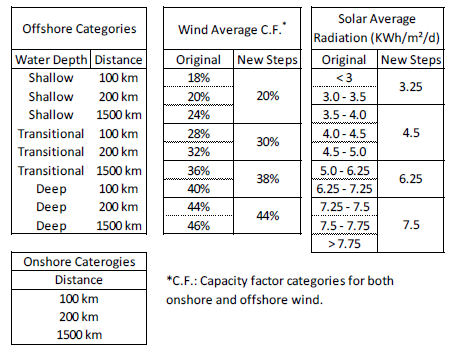# Non-biomass renewables - COFFEE-TEA

Corresponding documentation
Previous versions
Model information
Institution COPPE/UFRJ (Cenergia), Brazil, http://www.cenergialab.coppe.ufrj.br/.
Solution concept General equilibrium (closed economy)
Solution method The COFFEE model is solved through Linear Programming (LP). The TEA model is formulated as a mixed complementary problem (MCP) and is solved through Mathematical Programming System for General Equilibrium -- MPSGE within GAMS using the PATH solver.
Anticipation

Solar and Wind energy resources were estimated in the COFFEE model from 1 and other related engineering literature, especially for cost estimation. For solar resource the supply curve introduced in the model was based on solar radiance itself, instead of on electricity or power. Additionally, resources are split in four steps of increasing capacity factor.

For wind resources the capacity factor is split in offshore and onshore and the distance to major consumers and to the coastline are considered. This is important to generate better supply curves for energy resources, since the optimum decision from the model may be to use a lower capacity factor that is closer to the end consumer, depending on the costs associated. In the COFFEE model, wind resource was estimated considering 12 step curves for and 27 step discrete curves were created combining capacity factor, distance to shore and water depth for onshore and offshore, respectively. Figure 1 summarizes the wind and solar resource categories.Figure 1: Wind and solar resource categories

.

In the COFFEE model, the quality of the hydro resources derives from two major components: capacity factors and resource availability. These aspects are directly associated with exploitation costs. Thus, the total resources can be separated in terms of costs in order to create a supply curve for Hydro power. 2 and other related literature provide regional profiles for hydro projects and was used to estimate the hydro resource availability for the 18 regions of the model.Figure 2: Estimated installed capacity, total potential and potential by category for the hydro resource (GW).

.

1. ^  NREL(2015). National Renewable Energy Laboratory. Retrieved from https://www.nrel.gov/
2. ^ ireana2014